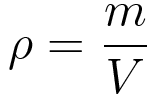# Density Converter

## How the Density Unit Converter works?

This simple and easy online density unit converter let you convert several types of density units. Select the unit to convert from in the left units list. Select the unit to convert to in the right units list. Enter the value to convert from into the source box on the left. The conversion result will immediately appear in the result box.

Conversions are performed by using a density conversion factor. By knowing the density conversion factor, converting between units can become a simple multiplication problem. Density itself is defined as how much mass a substance has in a specific volume.Usually, gases have a lower density than liquids because they have less cohesive particles, and these in turn less than solids. Although there are exceptions, usually increasing the temperature decreases density.